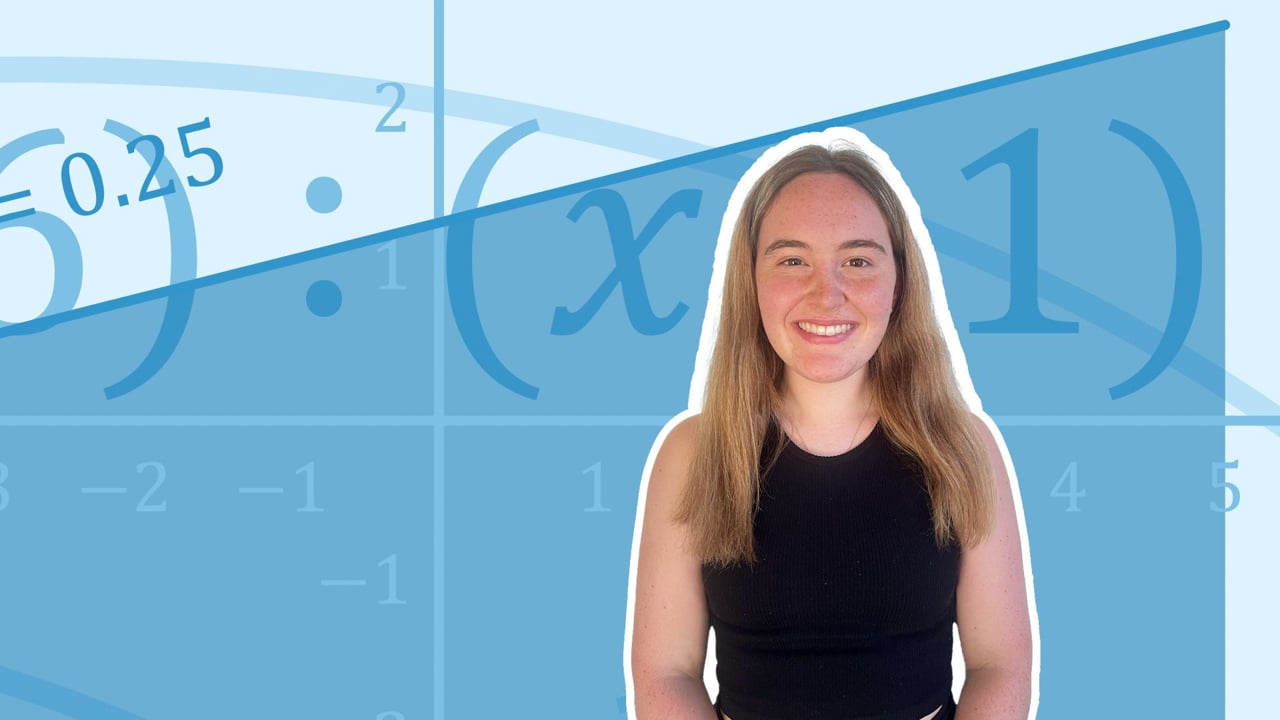Chapter overviewMaths

Number and place value

Multiplication and division

Fractions

Measurement

Geometry - properties of shapes

Geometry - position and direction

Statistics

Ratio and proportion

Algebra

Maths

# Missing number problems0%

Summary

# Missing number problems

## ​​In a nutshell

Expressions involving an unknown number are needed to solve missing number problems. Unknown numbers are usually represented by letters.

##### Example 1

$x + 10 = 12$

This is the same as asking: "what is added to $10$​ to get $12$​?"

The answer is $x=2$

Note: $x$ is the letter commonly used for an unknown.

## Method for solving

#### ​​Procedure

 1 Write out an equation 2 Work out the value of the unknown
##### Example 2

Anna has $£20$​. She shares $£5$​ with her brother and uses $£12$​ to buy a new dress. how much money does she have left?

Write an expression:

$5 + 12 + x = 20$

Solve for $x$:

$x= 3$

Anna has $\underline{£3}$​ remaining.

FAQs

• Question: How do I solve a missing number problem?

Answer: Use trial and error to try out possible solutions.

• Question: What do letters mean in an unknown number problem?

Answer: Unknown numbers are usually represented by letters.

Theory

Exercises